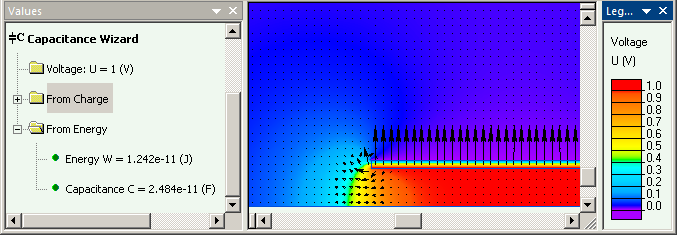QuickField

A new approach to field modelling
 Language: Global English Deutch Espanol Francais Italiano Danmark Ceske Chinese Pycckuü

Main >> Applications >> Sample problems >> Cylindrical capacitor

# Cylindrical capacitor

Capacitor consists of two coaxial cylindrical collinear electrodes. It may be simulated as 2D axisymmetric or 3D extrusion problem. Both approaches give same result and good correlation with analytical solution.

Problem Type:
2D axisymmetric/3D extrusion electrostatics.

Geometry:

a = 5 mm, b = 6 mm, L = 40 mm.

Given:
Relative permittivity of media ε = 2,
Voltage difference ΔU = 1 V.

Problem:
Find the capacitance of cylindrical capacitor.

Solution:
Analytical solution gives the capacitance value of ideal cylindrical capacitor (without end effects) as
C = 2πεε0 * l / ln(a/b) [F]. *

QuickField simulation gives energy W and electric potential U distribution. The capacitance then can be calculated as C = 2W / ΔU2.

Results:

Analytical solution (cylindrical capacitor without end effects):
C = 2*3.142*2*8.854e-12 * 0.04 / ln(0.006/0.005) = 0.4451e-11 / 0.1823 = 2.44e-11 F.

 Energy W Capacitance C 2D axisymmetrical simulation 1.24 pJ 2.48 pF 3D extrusion simulation 1.25 pJ 2.50 pF• Video: